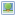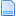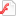## What Day Of The Week

2.5. What day of the week was 2 August 1953?
--------------------------------------------

To calculate the day on which a particular date falls, the following
algorithm may be used (the divisions are integer divisions, in which
remainders are discarded; % means all we want is the remainder):

a = (14 - month) / 12
y = year - a
m = month + 12*a - 2
For Julian calendar: d = (5 + day + y + y/4 + (31*m)/12) % 7
For Gregorian calendar: d = (day + y + y/4 - y/100 + y/400 + (31*m)/12) % 7

The value of d is 0 for a Sunday, 1 for a Monday, 2 for a Tuesday, etc.

Then I sat down and came up with this formula in order to calculate dates such as "The third Monday in January". I suspect these have been derived and written down somewhere by someone else; in any case, these formula are easy to derive, and useful for computing various holidays in electronic calendars.
First, let the above formula be called DoW(year,month,dayinmonth), which specifies that its arguements are the year (in numerical form), the month (1-12) and the day in the month (day number in month, 1-31).

In all the below formula, the following common-sense relation is used: -1%7 = 6; -2%7=5; .. -6%7=1, -7%7=0. Also, an N-day is a Sunday (N=0), through Saturday (N=6). The most generic formula is then:

Date In Month that is an N-day ON OR AFTER date Year-Month-Day =
Day + (N - DoW(Year,Month,Day))%7 .

Date In Month that is an N-day ON OR BEFORE date Year-Month-Day =
Day - (DoW(Year,Month,Day) - N)%7 .

These lead to quick formulae for determining the date of the first, second, third, fourth and fifth occurence of a Sunday, Monday, etc., in any particular month:

First N-day: N1 = 1 + (N - DoW(Year,Month,1))%7 ;
2nd N-day : N2 = 8 + (N - DoW(Year,Month,8))%7 ;
3rd N-day : N3 = 15 + (N - DoW(Year,Month,15))%7 ;
4th N-day : N4 = 22 + (N - DoW(Year,Month,22))%7 ;
5th N-day : N5 = 29 + (N - DoW(Year,Month,29))%7 .
(Note: Use common sense when trying to calculate the fifth N-day: check to see if the value you obtain is greater than the number of days in the month; if it is, the there is no fifth N-day in that month.)

Two visitors to this page, Timothy Barmann and Bobby Cossum, have independently suggested that the above five equations can be simplified into just one equation. Let Q be the occurence (first, second, third, fourth, fifth), and N will still represent the day of the week, as above. Then,
the Q-th N-day: NQ = 1 + (Q-1)*7 + (N - DoW(Year,Month,1))%7;
or equivalently
the Q-th N-day: NQ = 7*Q - 6 + (N - DoW(Year,Month,1))%7. So, to find the first Friday using the above equations, use Q=1, N=5; the third Monday is found using Q=3, N=1, etc.

In order to find, for example, the LAST Monday in a month, we need to know the length of the month; for all months except February, this is, of course, fixed. In any case, we have:

ND=Number of last day in month;
Last N-Day : NL = ND - (DoW(Year,Month,ND) - N)%7 .
Example: What date is the last Monday in May, 1996?

The last day in May is May 31, so ND=31.
Monday is what we want, so N=1
The day of the week of May 31, 1996 is found by following the first algorithm above: a=(14-5)/12=0
y=1996-a=1996-0=1996
m=5+0-2=3
d=(31+1996+499-19+4+(31*3)/12)%7= 5
So, May 31st is a Friday; then
NL=31-(5-1)%7=31-4=27
So, the last Monday in 1996 May is May 27.

### More Quizzes and Tests

1.11 Faces
2.12 Or 13
3.40 Seconds
4.A Good Test For Lateral Thinking
5.Are You In Love
6.Brain Exercise
7.Chocolate Maths 2001
8.Dalai Lama Personality Test
9.Dr Phil Psychology Test
10.Easiest Quiz In The World
11.Forest Personality Test
12.Geometric Puzzle
13.Get Out Your Pen And Paper
14.Get You Mind Outa Gutter
15.How Dumb You Are
16.How Smart Is Your Right Foot
17.Is Your Mind Normal
18.Just For Fun
19.Look At The Dot And Come Close
20.Magic Of Math
21.Murder Puzzle
22.Quick Eye Test
23.Seven Hearts
24.The Impossible Quiz
25.Tongue Twister
26.What Day Of The Week
27.What Do You See 1
28.What Do You See 2
29.What Do You See 3
30.What Others See In You
31.Where Is The Father